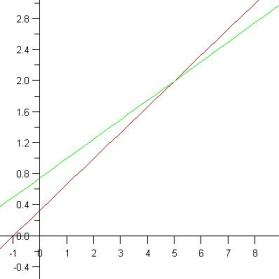# Linear systems – word problems

When it comes to using linear systems to solve word problems, the biggest problem is recognizing the important elements and setting up the equations. Once you do that, these linear systems are solvable just like other linear systems. The same rules apply. The best way to get a grip around these kinds of word problems is through practice, so we will solve a few examples here to get you accustomed to finding elements of linear systems inside of word problems.
Example 1:
A farmhouse shelters 16 animals. Some of them are chickens and the others are cows. Altogether these animals have 60 legs. How many chickens and how many cows are in the farmhouse?

First, to make the calculations clearer, we will choose symbols to represent the number of cows and the number of chickens. Let us say that the chickens will be represented with x and the cows with y.
Now, this task gave us enough information to make two equations. The first one is that the sum of the number of chickens (x) and the number of cows (y) is 16, since there are only 16 animals in the farmhouse. That equation should look like this:
x + y = 16

The second piece of information we have is that the total number of legs in the farmhouse is 60. Since we know that cows have four legs each and chickens have two legs each, we have enough information to make another equation. This one will look like this:
2*x + 4*y = 60

Now we have a system of linear equations with two equations and two variables. The only thing left to do now is to solve the system. We will solve it here for you, but if you need to remind yourself how to do that step by step, read the article called Systems of linear equations.
x = 16 – y
32 – 2y + 4y = 60
2y = 28
y = 14
x = 2

We can now see that there are two chickens and 14 cows in the farmhouse. The next example will be a bit harder,

Example 2:
Rodney’s Kitchen Supplies makes and sells spoons and forks. It costs the store \$2 to buy the supplies needed to make a fork, and \$1 for the supplies needed to make a spoon. The store sells the forks for \$4 and the spoons for 5\$. Last month Rodney’s Kitchen Supplies spent \$39 on supplies and sold the all of the forks and spoons that were made last month using those supplies for \$93. How many forks and spoons did they make?

As we did in the first example, we will first designate symbols to available variables. So, the number of forks made will be represented with x and the number of spoons with y. Again, we have enough information to make two equations.
The total cost of making a particular number of forks (x), which cost \$2 to make each, and a particular number of spoons (y), which cost \$1 to make each, is \$39. So that will be our first equation and it will look like this:
2*x + y = 39
The other piece of information tells us that if we sell that number of forks (x) for \$4 each and that number of spoons (y) for \$5 each, we will make \$93. And that will be our second equation:
4*x + 5*y = 93
This was the hard part. Now all we have to do is to solve this linear system to find how many spoons and how many forks did we make last month.
y = 39 – 2x
4x + 5*(39 – 2x) = 93
4x + 195 – 10x = 93
-6x = 93 – 195
-6x = -102 |: (-6)
x = 17
y = 5

We can see that last month the store made and sold 17 forks and five spoons.Although they can seem complicated, mastery and understanding of linear systems and associated word problems will come with a bit of practice. With experience you will be able to recognize their elements and solve even complicated systems with ease. Feel free to use the math worksheets below to practice solving this type of linear systems.

## Linear systems – word problems exams for teachers

 Exam Name File Size Downloads Upload date Linear systems – Word problems – very easy1 16 kB 13748 February 27, 2012 Linear systems – Word problems – very easy2 16 kB 7514 February 27, 2012 Linear systems – Word problems – easy1 18 kB 10450 February 27, 2012 Linear systems – Word problems – easy2 17 kB 6330 February 27, 2012 Linear systems – Word problems – medium1 20 kB 15064 February 27, 2012 Linear systems – Word problems – medium2 22 kB 7919 February 27, 2012 Linear systems – Word problems – hard1 23 kB 9776 February 27, 2012 Linear systems – Word problems – hard2 23 kB 5030 February 27, 2012 Linear systems – Word problems – very hard1 26 kB 6846 February 27, 2012 Linear systems – Word problems – very hard2 26 kB 5998 February 27, 2012

## Linear systems – word problems worksheets for studets

 Worksheet Name File Size Downloads Upload date Linear systems – Word problems – Integers 366.5 kB 14811 September 3, 2019 Linear systems – Word problems – Decimals 380.8 kB 13278 September 3, 2019